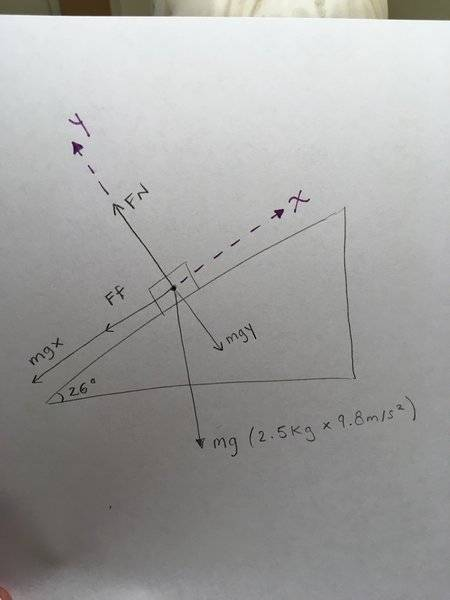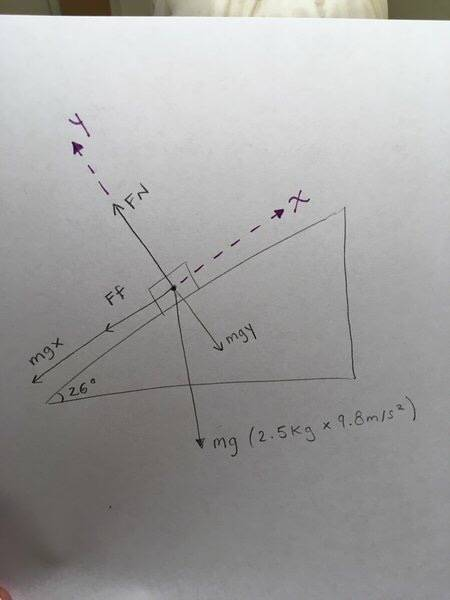# Work Problem - Distance of particle moving up an incline

shmoop

## Homework Statement

Hi! I'm having issues with this practice problem. Any advice on what I'm doing wrong would help!!!

A 2.5 kg particle is projected with an initial speed of 3.3m/s along a surface for which the coefficient of friction is 0.7. Find the distance it travels given that the particle moves up a 26 degree incline.

W=ΔKE
W=F*d
Ff=μFn
W=ΔKE+ΔPE
KE=1/2mv2
PE=mgh

## The Attempt at a Solution

mg=2.5kg*-9.8m/s2=-24.5N

-24.5Nsin26=-10.74 N = mgx
-24.5Ncos26=-22.02N = mgy

ΣFy=0=Fn-mgy
Fn= 22.02 N

Ff = Fnμ
Ff = 22.02N*0.7
Ff=15.14 N

W= KEf-KEf+PEf-PEi
W=.5mv2-.5mv2+mgh-mgi
W=0.5*0.3kg*(3.3m/s)2-0+2.5kg*9.8*h-0
W=F*d

Here I assumed KEf was 0 because the final velocity is 0, and I assumed PEi was 0 because the inital height is 0.

The forces applied that aren't in equilibrium are mgx, and Ff.

W= (-15.14N-10.74N)*d

-26.15N*d=0.5*0.3kg*(3.3m/s)2-0+2.5kg*9.8*h

So instead of having 2 unknown variables, I made h equal to...

h= d*sin26

So.. I inserted that in the previous equation:

-26.15N*d=0.5*0.3kg*(3.3m/s)2-0+2.5kg*9.8*d*sin26

Factored out d...

d(-26.15+24.5sin26)=-13.6125

d=-13.6125/(-26.15+24.5sin16)
d=0.88m

And this answer is incorrect. I am unsure where I went wrong... Any thoughts? Thank you!!!

For an image of what my free body diagram looks like, I posted it below!!

Last edited by a moderator:

-24.5Nsin26=-10.74 N = mgx
-24.5Ncos25=-22.02N = mgy

ΣFy=0=Fn-mgy
Fn= 22.02 N
I'm not really sure what you are doing here. You have effectively already calculated the normal force here
-24.5Ncos25=-22.02N = mgy
although the angle is wrong. It is also unclear why you are setting this equal to mgy.

•shmoop
shmoop
I'm not really sure what you are doing here. You have effectively already calculated the normal force here
-24.5Ncos25=-22.02N = mgy
although the angle is wrong. It is also unclear why you are setting this equal to mgy.

Hi! Thanks for taking the time to answer.
The 25 degrees was a typo, I did use 26 degrees to calculate mgy.
I calculated the horizontal gravitational force, mgx, as I believe (its force applied to the object + the friction force) multiplied by the distance, are equal to work.
A.k.a. the applied forces to the object which are not in equilibrium (and the x axis is not in equilibrium)

Is that an incorrect assumption? If so, any pointers?

shmoop
I'm not really sure what you are doing here. You have effectively already calculated the normal force here
-24.5Ncos25=-22.02N = mgy
although the angle is wrong. It is also unclear why you are setting this equal to mgy.

I was calculating the gravitational force, the mgy = gravitational force along the y (perpendicular to the incline)
I then made the value positive later and said that it was equal to the normal (Fn). Because ΣFy=0

SammyS
Staff Emeritus
Homework Helper
Gold Member

## Homework Statement

Hi! I'm having issues with this practice problem. Any advice on what I'm doing wrong would help!!!

A 2.5 kg particle is projected with an initial speed of 3.3m/s along a surface for which the coefficient of friction is 0.7. Find the distance it travels given that the particle moves up a 26 degree incline.

W=ΔKE
W=F*d
Ff=μFn
W=ΔKE+ΔPE
KE=1/2mv2
PE=mgh

## The Attempt at a Solution

mg=2.5kg*-9.8m/s2=-24.5N

-24.5Nsin26=-10.74 N = mgx
-24.5Ncos26=-22.02N = mgy

ΣFy=0=Fn-mgy
Fn= 22.02 N

Ff = Fnμ
Ff = 22.02N*0.7
Ff=15.14 N

W= KEf-KEf+PEf-PEi
W=.5mv2-.5mv2+mgh-mgi
W=0.5*0.3kg*(3.3m/s)2-0+2.5kg*9.8*h-0
W=F*d

Here I assumed KEf was 0 because the final velocity is 0, and I assumed PEi was 0 because the inital height is 0.

The forces applied that aren't in equilibrium are mgx, and Ff.

W= (-15.14N-10.74N)*d

-26.15N*d=0.5*0.3kg*(3.3m/s)2-0+2.5kg*9.8*h

So instead of having 2 unknown variables, I made h equal to...

h= d*sin26

So.. I inserted that in the previous equation:

-26.15N*d=0.5*0.3kg*(3.3m/s)2-0+2.5kg*9.8*d*sin26

Factored out d...

d(-26.15+24.5sin26)=-13.6125

d=-13.6125/(-26.15+24.5sin16)
d=0.88m

And this answer is incorrect. I am unsure where I went wrong... Any thoughts? Thank you!!!

Using a free-body-diagram would be helpful.

shmoopshmoop

Using a free-body-diagram would be helpful.SammyS
Staff Emeritus
Homework Helper
Gold Member
You are using two different expressions for work.

The net work is given by Wnet = Fnet⋅d ,
and is related to the change in kinetic energy by: Wnet = ΔKE .

The work done by non-conservative forces is related to the change in PE and KE by: Wnon-con = ΔKE+ΔPE .

You were using these interchangeably, and therefore accounted gravity's effect twice.

...
2. Homework Equations

W=ΔKE
W=F*d
Ff=μFn
W=ΔKE+ΔPE
KE=1/2mv2
PE=mgh

## The Attempt at a Solution

...

W= KEf-KEf+PEf-PEi
W=.5mv2-.5mv2+mgh-mgi
W=0.5*0.3kg*(3.3m/s)2-0+2.5kg*9.8*h-0
W=F*d
The above is true for the work done by non-conservative forces. The PE accounts for effects of gravity.

The following gives the net Force and thus the net Work.
...

The forces applied that aren't in equilibrium are (mg)x, and Ff.

W= (-15.14N-10.74N)*d

-26.15N*d=0.5*0.3kg*(3.3m/s)2-0+2.5kg*9.8*h
That last equation has effects of gravity taken into account twice.

•shmoop
shmoop
You are using two different expressions for work.

The net work is given by Wnet = Fnet⋅d ,
and is related to the change in kinetic energy by: Wnet = ΔKE .

The work done by non-conservative forces is related to the change in PE and KE by: Wnon-con = ΔKE+ΔPE .

You were using these interchangeably, and therefore accounted gravity's effect twice.

The above is true for the work done by non-conservative forces. The PE accounts for effects of gravity.

The following gives the net Force and thus the net Work.

That last equation has effects of gravity taken into account twice.

Ohhh! So.. Work will be

Wnet=Fnet*d

and Fnet will only be equal to that of the frictional force?

shmoop
You are using two different expressions for work.

The net work is given by Wnet = Fnet⋅d ,
and is related to the change in kinetic energy by: Wnet = ΔKE .

The work done by non-conservative forces is related to the change in PE and KE by: Wnon-con = ΔKE+ΔPE .

You were using these interchangeably, and therefore accounted gravity's effect twice.

The above is true for the work done by non-conservative forces. The PE accounts for effects of gravity.

The following gives the net Force and thus the net Work.

That last equation has effects of gravity taken into account twice.

Using the previous equation, I have tried this instead:

Fnet=-15.41 N

Fnet*d=½(0.3kg)(3.3m/s)2+(2.5kg)(9.8m/s2)(dsin26°)

d(-15.41N+(2.5kg)(9.8m/s2))=½(0.3kg)(3.3m/s)2

d=½(0.3kg)(3.3m/s)2/((-15.41N+(2.5kg)(9.8m/s2))

d=2.91m

This is incorrect as well. :( Any further pointers? Thank you for your time, effort, and knowledge!!

and Fnet will only be equal to that of the frictional force?
No, the gravitational force is still there.

Both friction and gravity do work here. You know both of these forces and the initial kinetic energy. So how much work is required by these forces to bring the kinetic energy to zero?

•shmoop
SammyS
Staff Emeritus
Homework Helper
Gold Member
The net Force: That's the sum of all the forces not in equilibrium. You previously had that correct. Use this for net Work, Wnet.
Work - Energy Theorem: Wnet = ΔKE.​

Otherwise, use the WN.C. work done only by non-conservative forces.
The only non-conservative force here is friction.
WN.C. = ΔKE+ΔPE​

It appears that you keep mixing these two this together ..

shmoop
The net Force: That's the sum of all the forces not in equilibrium. You previously had that correct. Use this for net Work, Wnet.
Work - Energy Theorem: Wnet = ΔKE.​

Otherwise, use the WN.C. work done only by non-conservative forces.
The only non-conservative force here is friction.
WN.C. = ΔKE+ΔPE​

It appears that you keep mixing these two this together ..

Oh. So I should do this..?

Wnet=F*d

F being friction and mgx

And then:

F*d=0-1/2mvi^2

Since KEf is 0??

(Thanks again)

shmoop
No, the gravitational force is still there.

Both friction and gravity do work here. You know both of these forces and the initial kinetic energy. So how much work is required by these forces to bring the kinetic energy to zero?

Hi, thanks for popping in to help.

So does this make more sense?

Wnet=F*d

F being friction and mgx

And then:

F*d=0-1/2mvi^2

Since KEf is 0??

(Thanks)

SammyS
Staff Emeritus
Homework Helper
Gold Member
Using the previous equation, I have tried this instead:

Fnet=-15.41 N

Fnet*d=½(0.3kg)(3.3m/s)2+(2.5kg)(9.8m/s2)(dsin26°)

d(-15.41N+(2.5kg)(9.8m/s2))=½(0.3kg)(3.3m/s)2

d=½(0.3kg)(3.3m/s)2/((-15.41N+(2.5kg)(9.8m/s2))

d=2.91m

This is incorrect as well. :( Any further pointers? Thank you for your time, effort, and knowledge!!
Other than mislabeling and some sign errors this should work.

What you call Fnet here is just the friction force: That's the Non-Conservative force, not the net force. The work done by this force is equal to the change in KE+PE .

You messed up the change in KE. Final minus Initial is negative, Right?

Finally, you have an Algebra error: added PE rather than subtracting .

So does this make more sense?

Wnet=F*d

F being friction and mgx

And then:

F*d=0-1/2mvi^2

Since KEf is 0??
Yes

•shmoop
shmoop
Other than mislabeling and some sign errors this should work.

What you call Fnet here is just the friction force: That's the Non-Conservative force, not the net force. The work done by this force is equal to the change in KE+PE .

You messed up the change in KE. Final minus Initial is negative, Right?

Finally, you have an Algebra error: added PE rather than subtracting .

Thank you!! It is getting late where I am, but I will try this in the morning.

Thank you x 10000.

shmoop
Yes

This solved my problem!! THANK YOU

SammyS
Staff Emeritus
Homework Helper
Gold Member
This solved my problem!! THANK YOU
So, what are those last few steps as well as you final answer ?

(We like feedback.)

shmoop
So, what are those last few steps as well as you final answer ?

(We like feedback.)

This is what I did, based on my conversation with Nfuller

Wnet=f*d

f=Ff+mgx

Ff+mgx=-1/2mvi^2

(-15.14N-10.74N)*d=-(1/2)(2.5kg)(3.3m/s)^2

-25.88N*d=-13.61

d=0.52m